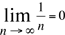Name: Evan Who is asking: Student Level of the question: Secondary Question: I was thinking the other day when i was in math class that when you divide 1 by say n you'll get 1/n. As the value of n increases the smaller the number you get. So if you divide 1/infinity would that equal zero? And if that is true then would 1/0=infinity be true also? Hi Evan, Your observation that As the value of n increases the closer 1/n gets to zero. is correct and is a very important idea but I don't like writing 1/infinity. The arithmetic operations apply to numbers and infinity is not a number so I don't like the idea of trying to divide by something that is not a number. Nevertheless I would like a more mathematical way to say As the value of n increases the closer 1/n gets to zero. To do this mathematicians use the idea of a limit, which is the fundamental concept of calculus, and say that the limit of 1/n as n approaches infinity is zero, and write this statementIf you apply the same idea to try to evaluate 1/0, that is you ask As the value of n gets close to zero what happens to the value of 1/n? I am thinking of n as a positive number. If you try this you realize that as n gets close to zero, 1/n gets larger and larger and doesn't approach any finite value so I might say The limit of 1/n as n approaches zero is infinity. or what I prefer to say is that The limit of 1/n as n approaches zero does not exist. As n approaches zero, 1/n just doesn't approach any numeric value. You can find another approach to attempting to evaluate 1/0 in the answer to a previous question. Penny